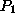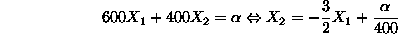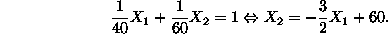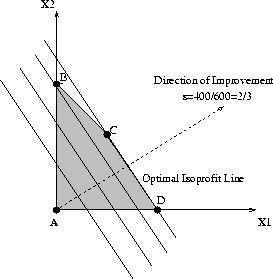Next: Infeasible 2-var LP's Up: Graphical solution of 2-var Previous: Graphical solution of the

#### 2-var LP's with many optimal solutions

Consider our prototype example with the unit profit of itembeing \$600 instead of \$200. Under this modification, the problem isoprofit lines become:and they are parallel to the line corresponding to the first problem constraint:Therefore, if we try to apply the optimizing technique of the previous paragraph in this case, we get the situation depicted below (Figure 4), i.e., every point in the line segment CD is an optimal point, providing the optimal objective value of \$24,000.Figure 4: An LP with many optimal solutions

It is worth-noticing that even in this case of many optimal solutions, we have two of them corresponding to ``corner'' points of the feasible region, namely points C and D.

UAL Data
Fri Jun 20 15:03:05 CDT 1997